### IMO Shortlist 1984 problem 9

Kvaliteta:
Avg: 0,0
Težina:
Avg: 0,0
Let$a, b, c$ be positive numbers with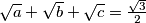$\sqrt a +\sqrt b +\sqrt c = \frac{\sqrt 3}{2}$. Prove that the system of equations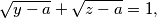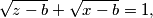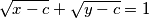has exactly one solution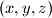$(x, y, z)$ in real numbers.
Izvor: Međunarodna matematička olimpijada, shortlist 1984